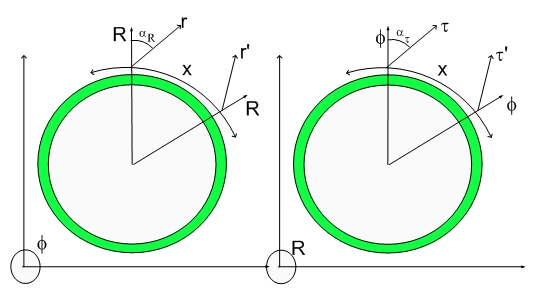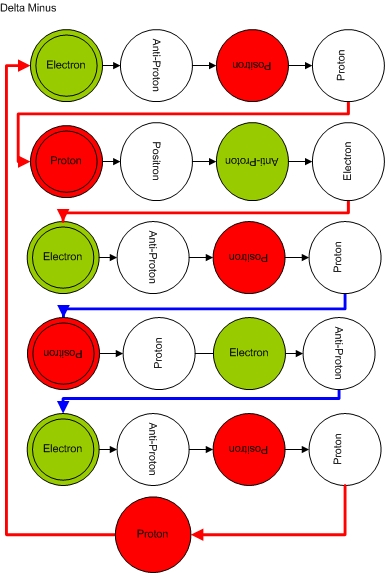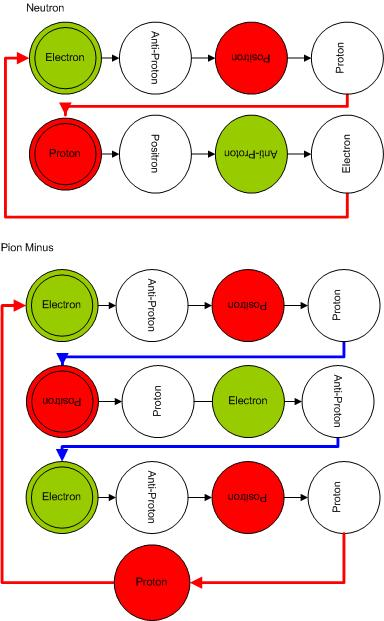# The Twisted Deltas - Delta MinusThe Twisted Deltas - Delta Minus

Here is the Delta Minus:It decays into a Neutron and a Pion Minus...:) ...Just rearrange the red lines on the Delta Minus Balls Diagram.

Different spin arrangements will lead to a total spin 1/2 or 3/2 (Sigma Minus or Delta Minus) moieties.

Notice that the Delta Minus Dimensional Multichord contains exactly two Blue Transmultation Transitions (blue lines = electron-positron or positron-electron transmutations) and four Red Transmutations Transitions (red lines= electron-proton or proton-electron transmutations, thus resulting in the same mass as the other Deltas. Similar statement is valid for the Sigmas.

Notice that a Pion Minus is a trimeric moiety, that is, since one cannot know which phase of the electron-positron-electron chords is in phase with the Universe, the correct representation is a linear combination of states with 1/3 probability each. This means that one can think about a particle either a having a longer cycle or having smaller mass per cycle. I used a one atomic mass unit Fat Electron and a cycle equal to twice the cycle of an hydrogen atom (neutral zero spin matter). I could had chosen to state the Fat Electron not as Fat, just half a.m.u and then use a dimeric representation and the standard cycle. The results would be the same.

Any way one should pay attention to multichords and which representation one chooses

There are no alternative decay channel for the Delta Minus...:)

Please feel free to ask any questions. I am trying not to be repetitive when visiting similar particles.

Cheers,

MP

PS - Since Pions Plus/Minus are trimeric, Delta Plus/Minus are pentameric, etc...This reflection into the first chord also means that the particle gains a moment of inertia, helicity and pseudo-rotates as one travels along R.
Currently unrated

### New Comment

required

required (not published)

optional

required